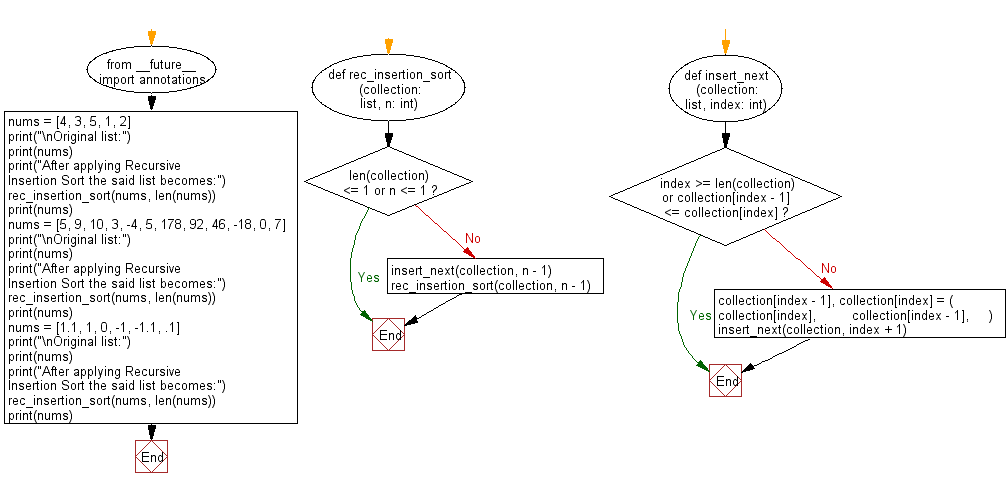﻿ Python: Sort a given collection of numbers and its length in ascending order using Recursive Insertion Sort - w3resource# Python: Sort a given collection of numbers and its length in ascending order using Recursive Insertion Sort

## Python Search and Sorting : Exercise-29 with Solution

Write a Python program to sort a given collection of numbers and its length in ascending order using Recursive Insertion Sort.

Sample Solution:

Python Code:

``````#Ref.https://bit.ly/3iJWk3w
from __future__ import annotations

def rec_insertion_sort(collection: list, n: int):
# Checks if the entire collection has been sorted
if len(collection) <= 1 or n <= 1:
return
insert_next(collection, n - 1)
rec_insertion_sort(collection, n - 1)
def insert_next(collection: list, index: int):
# Checks order between adjacent elements
if index >= len(collection) or collection[index - 1] <= collection[index]:
return
# Swaps adjacent elements since they are not in ascending order
collection[index - 1], collection[index] = (
collection[index],
collection[index - 1],
)
insert_next(collection, index + 1)
nums = [4, 3, 5, 1, 2]
print("\nOriginal list:")
print(nums)
print("After applying Recursive Insertion Sort the said list becomes:")
rec_insertion_sort(nums, len(nums))
print(nums)
nums = [5, 9, 10, 3, -4, 5, 178, 92, 46, -18, 0, 7]
print("\nOriginal list:")
print(nums)
print("After applying Recursive Insertion Sort the said list becomes:")
rec_insertion_sort(nums, len(nums))
print(nums)
nums = [1.1, 1, 0, -1, -1.1, .1]
print("\nOriginal list:")
print(nums)
print("After applying Recursive Insertion Sort the said list becomes:")
rec_insertion_sort(nums, len(nums))
print(nums)
```
```

Sample Output:

```Original list:
[4, 3, 5, 1, 2]
After applying Recursive Insertion Sort the said list becomes:
[1, 2, 3, 4, 5]

Original list:
[5, 9, 10, 3, -4, 5, 178, 92, 46, -18, 0, 7]
After applying Recursive Insertion Sort the said list becomes:
[-18, -4, 0, 3, 5, 5, 7, 9, 10, 46, 92, 178]

Original list:
[1.1, 1, 0, -1, -1.1, 0.1]
After applying Recursive Insertion Sort the said list becomes:
[-1.1, -1, 0, 0.1, 1, 1.1]
```

Flowchart:Python Code Editor:

What is the difficulty level of this exercise?

Test your Programming skills with w3resource's quiz.

﻿

## Python: Tips of the Day

Use Reversed() In for Loops:

```>>> tasks = ['laundry', 'picking up kids', 'gardening', 'cooking']